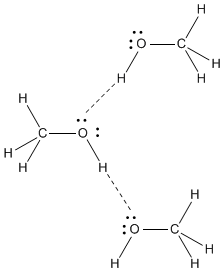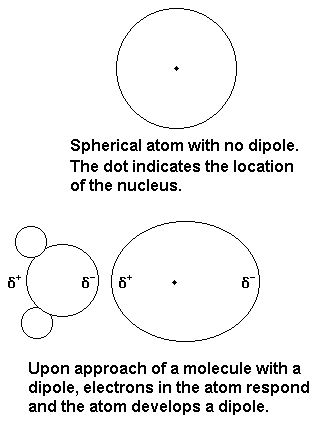# What is the strongest intermolecular force? Identify the strongest intermolecular forces in methanol and carbon tetrachloride. How do your answers explain what happens when you add methanol to carbon tetrachloride?

Sep 7, 2017

Well intermolecular hydrogen bonding operates in methanol.....

#### Explanation:

And only dispersion forces operate in carbon tetrachloride. We might assume that the latter is more volatile than methanol. But as physical scientists, we should consider the data, and the best metric of intermolecular attraction should be normal boiling point...

$\text{boiling point of methanol} = 64.7$ ""^@C

$\text{boiling point of carbon tetrachloride} = 76.7$ ""^@C

$\text{boiling point of ethanol} = 78.4$ ""^@C

So in fact carbon tet is LESS volatile than methanol. Mind you it is a much heavier molecule than methanol, with more electrons, and thus greater opportunity for dispersion forces. A better molecule for comparison might be chloromethane, or methylene chloride....

$\text{boiling point of chloromethane} = - 24.2$ ""^@C

$\text{boiling point of methylene chloride} = 39.6$ ""^@C

Are you happy with this rationale?

PS I do not even know whether methanol is soluble in carbon tet. Is it? This is the province of experiment. Certainly, methanol is INSOLUBLE in hexane, $n - {C}_{6} {H}_{14}$. Why should this be so?

Sep 8, 2017

The strongest intermolecular force is a dipole-induced dipole interaction.

#### Explanation:

Intermolecular forces

The three most common intermolecular forces, from strongest to weakest are:

• dipole - dipole (which includes hydrogen bonds)
• dipole - induced dipole
• London dispersion forces

Intermolecular forces in methanol

The strongest intermolecular forces in methanol are hydrogen bonds ( an especially strong type of dipole-dipole interaction).Intermolecular forces in ${\text{CCl}}_{4}$

The $\text{C-Cl}$ bonds are polar but, because of the tetrahedral symmetry, the bond dipoles cancel each other.Thus, ${\text{CCl}}_{4}$ is a nonpolar molecule, and its strongest intermolecular forces are London dispersion forces.

$\text{CH"_3"OH}$ in ${\text{CCl}}_{4}$

$\text{CH"_3"OH}$ is not very soluble in ${\text{CCl}}_{4}$, but some molecules of $\text{CH"_3"OH}$ can manage to get into the ${\text{CCl}}_{4}$.

We tend to think of ${\text{CCl}}_{4}$ as a spindly molecule with four spokes coming out of a central carbon atom.

However, the electron cloud around the molecule is nearly spherical, as in the diagram above.

The major interaction between $\text{CH"_3"OH}$ and ${\text{CCl}}_{4}$ will be dipole-induced dipole interactions, in which the $\text{O-H}$ bond dipole induces a dipole in the nonpolar ${\text{CCl}}_{4}$.